Ex 14.1

Chapter 14 Class 10 Statistics
Serial order wise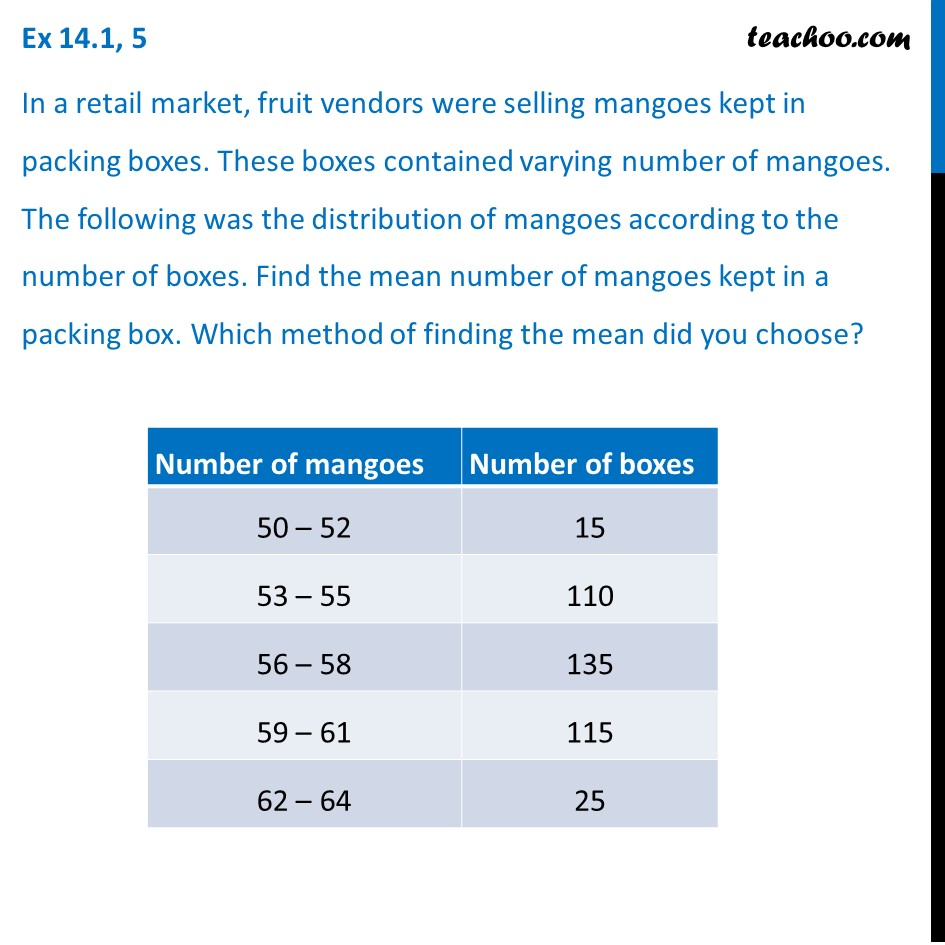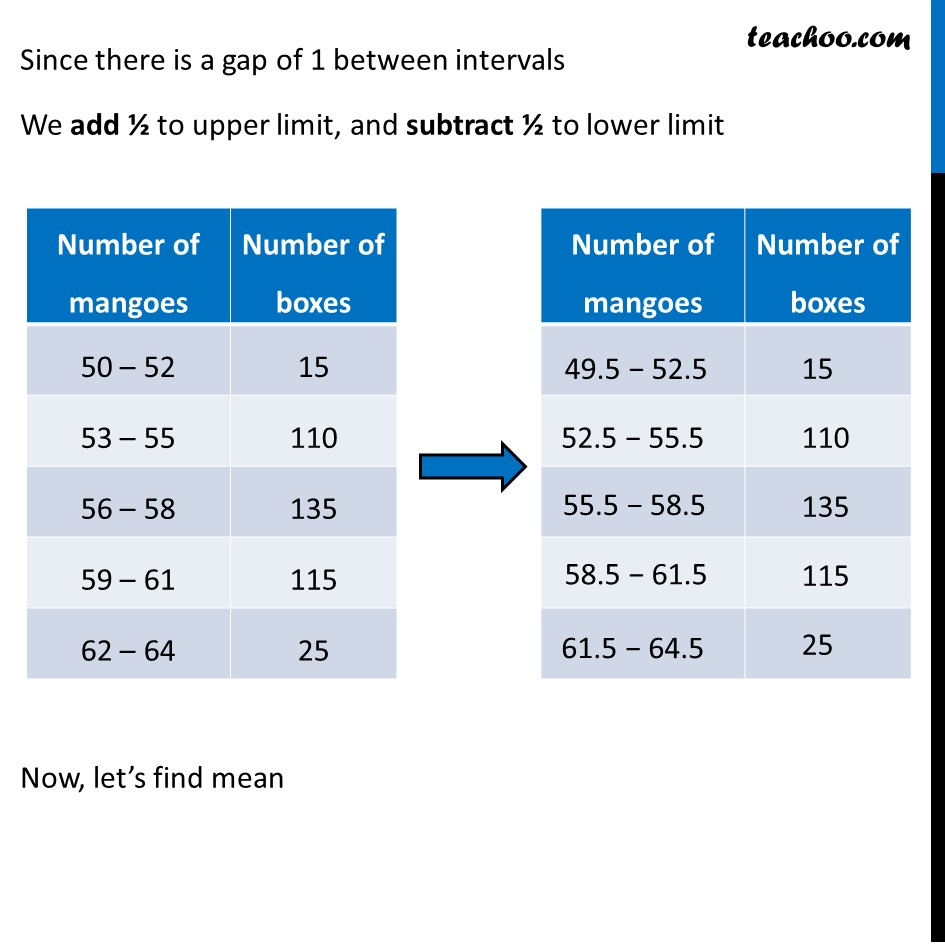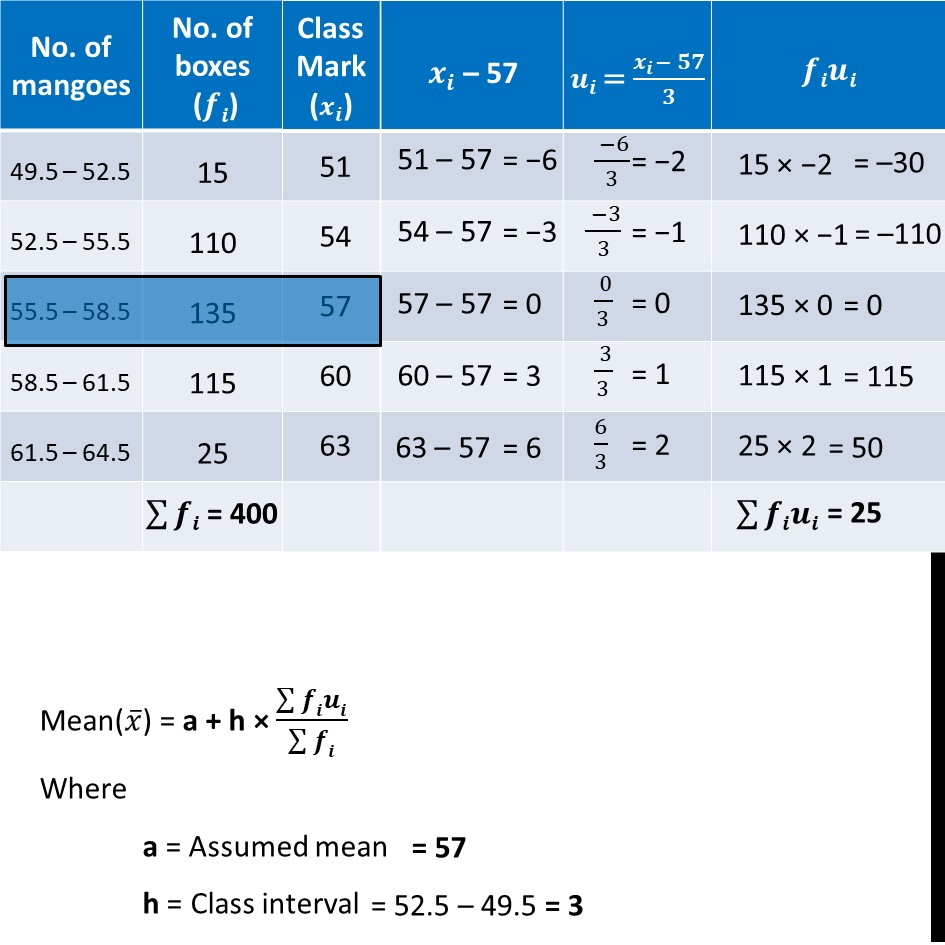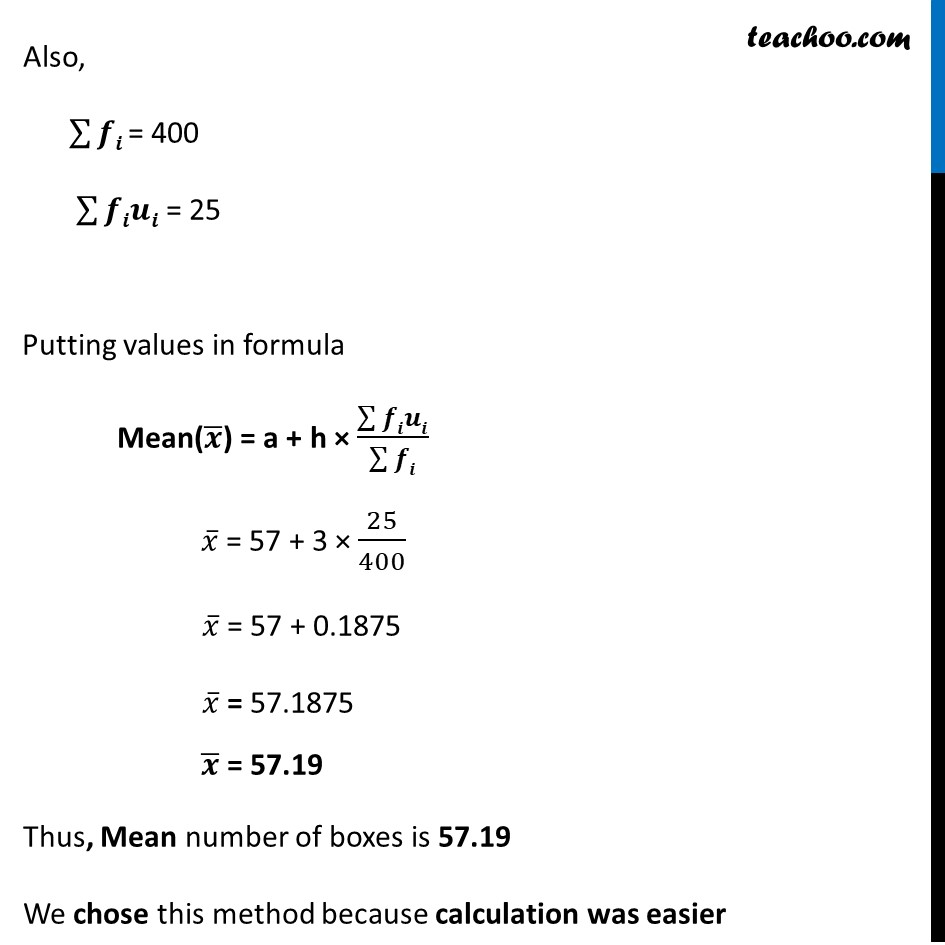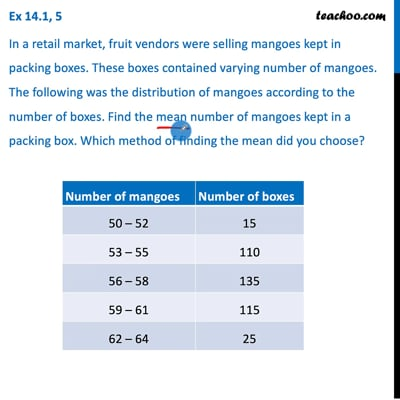This video is only available for Teachoo black users

Solve all your doubts with Teachoo Black (new monthly pack available now!)

### Transcript

Ex 14.1, 5 In a retail market, fruit vendors were selling mangoes kept in packing boxes. These boxes contained varying number of mangoes. The following was the distribution of mangoes according to the number of boxes. Find the mean number of mangoes kept in a packing box. Which method of finding the mean did you choose? Since there is a gap of 1 between intervals We add ½ to upper limit, and subtract ½ to lower limit Now, let’s find mean Mean(𝑥 ̅) = a + h × (∑▒𝒇𝒊𝒖𝒊)/(∑▒𝒇𝒊) Where a = Assumed mean h = Class interval = 57 = 52.5 – 49.5 = 3 Also, ∑▒𝒇𝒊 = 400 ∑▒𝒇𝒊𝒖𝒊 = 25 Putting values in formula Mean(𝒙 ̅) = a + h × (∑▒𝒇𝒊𝒖𝒊)/(∑▒𝒇𝒊) 𝑥 ̅ = 57 + 3 × 25/400 𝑥 ̅ = 57 + 0.1875 𝑥 ̅ = 57.1875 𝒙 ̅ = 57.19 Thus, Mean number of boxes is 57.19 We chose this method because calculation was easier